American Journal of Modern Physics
Volume 5, Issue 1-1, February 2016, Pages: 33-39

The Painlevé-Gullstrand ‘Extension’ - A Black Hole Fallacy

Stephen J. Crothers

Alpha Institute of Advanced Study, Brisbane, Australia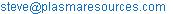Stephen J. Crothers. The Painlevé-Gullstrand ‘Extension’ – A Black Hole Fallacy. American Journal of Modern Physics. Special Issue: Physics Without Higgs and Without Supersymmetry. Vol. 5, No. 1-1, 2016, pp. 33-39. doi: 10.11648/j.ajmp.s.2016050101.15

Abstract: A number of methods have been employed by cosmologists to effect what they call an ‘extension’ of their ‘Schwarzschild solution’, to remove the singularity at their ‘Schwarzschild radius’ rs = 2Gm/c^2; the latter they maintain is the radius of the ‘event horizon’ of a black hole. They call the singularity at the Schwarzschild radius a coordinate singularity. The method of extension most often employed by cosmologists is the Kruskal-Szekeres extension, but sometimes the Painlevé-Gullstrand extension is used. The quantity r appearing in all these metrics is invariably treated by cosmologists as the radial distance, most evident in their ‘Schwarzschild radius’. Intuitively, radial distance is ≥ 0 and so, on their false assumption that r is the radial distance in the ‘Schwarzschild solution’, the cosmologists seek to drive it down to zero where they say there is a physical singularity. Although cosmologists have devised mathematical-like methods to seemingly do this, to produce their black hole, all their methods violate the rules of pure mathematics and so they are inadmissible. Consequently, the Painlevé-Gullstrand ‘extension’ is invalid. Moreover, since material sources cannot be both present in and absent from Einstein’s field equations by the very same mathematical constraint, the whole theory of black holes is fallacious.

Keywords:General Relativity, Black Hole, Metric Extensions, Ricci Tensor, Escape Velocity

Contents

1. Introduction

The methods employed by cosmologists to extend the so-called ‘Schwarzschild solution’ to remove the singularity at the ‘Schwarzschild radius’ rs = 2Gm/c2 for a smooth descent to a supposed physical singularity at r = 0 include the following:

1.   Eddington-Finkelstein coordinates

2.   Lemaȋtre coordinates

3.   Kruskal-Szekeres coordinates

4.   Painlevé-Gullstrand coordinates

I have proven elsewhere [1-5] that the first three methods do not extend Schwarzschild’s solution to produce a black hole. I provide herein the proof that the Painlevé-Gullstrand coordinates do not do so either, although it is a simple consequence of the invalidity of the first three methods.

2. Schwarzschild’s Solution

Schwarzschild’s solution is that for Einstein’s supposed field equations in the absence of matter, Rμν = 0. Consider

Schwarzschild’s solution1 ,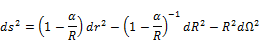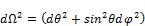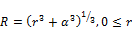(1)

The term α is a positive real-valued constant and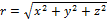. The metric ds2 is singular only at r = 0. Note that neither r nor R is the radius of anything in (1). Neither R nor r are even distances in the metric. Since R is not the radius there is no a priori reason to suppose that it must go down to zero, and hence there is no a priori reason to suppose that r must go down to −α. Schwarzschild’s solution is static, spherically symmetric, and asymptotically flat.

Consider next Droste’s solution2 ,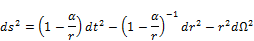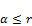(2)

The term α is a positive real-valued constant. The metric ds2 is singular only at r = α. Note that r is not the radius of anything; it is not even a distance in the metric. Since r is not the radius there is no a priori reason to suppose that it must go down to zero. Droste’s solution is equivalent to Schwarzschild’s [1-5,7].

Consider now Hilbert's solution ,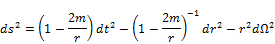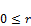(3)

Here the speed of light and Newton’s universal constant of gravitation are set to unity (c = 1, G = 1). If c and G are included explicitly, Hilbert’s solution is,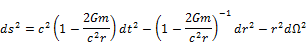(3b)

from which it can be seen that the Newtonian expression for escape speed has been inserted post hoc, by examining the ‘Schwarzschild radius’,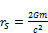(3c)

which when solved for c gives,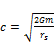(3d)

It is from equations (3c) and (3d) that the cosmologists obtain their ‘radius’ of their ‘event horizon’ and their ‘escape speed’ at their ‘event horizon’ .

"black hole A region of spacetime from which the escape velocity exceeds the velocity of light. In Newtonian gravity the escape velocity from the gravitational pull of a spherical star of mass M and radius R is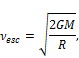where G is Newton’s constant. Adding mass to the star (increasing M), or compressing the star (reducing R) increases vesc. When the escape velocity exceeds the speed of light c, even light cannot escape, and the star becomes a black hole. The required radius RBH follows from setting vesc equal to c: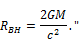The term m in (3) and (3b) is a positive real-valued constant representing mass, as revealed explicitly in the quote above ; the source of a gravitational field supposedly described by Hilbert’s solution. According to cosmologists Hilbert’s metric is singular at r = 2m (r = 2Gm/c2) and at r = 0. Note that r is not the radius of anything in Hilbert’s solution; it is not even a distance in the metric [1-4]. Since r is not the radius there is no a priori reason to suppose that it must go down to zero. It is asserted by cosmologists that r = 2m (r = 2Gm/c2) is the radius of a black hole event horizon - their ‘Schwarzschild radius’, which is a place of no exit. Things can pass the event horizon going inwards but nothing can even leave the event horizon trying to go outwards, and nothing can emerge from below it. It is from Hilbert’s solution that the black hole was first conjured. Hilbert’s solution is not equivalent to Schwarzschild’s, on account of its 0 ≤ r. Note that Hilbert’s solution is a corruption of Droste’s solution in that Hilbert set α = 2m and set 0 ≤ r, and thereby invented the black hole.

3. Infinite Equivalence Class

The quantity r in Hilbert’s solution can be replaced by any analytic function of r and the resulting metric will still possess spherical symmetry and will still satisfy Rμν = 0. However, not any analytic function will do. For instance, replace Hilbert’s r with er, and take into account his alleged 0 ≤ r. The result is,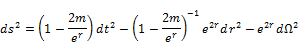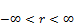(4)

This metric is singular only at r = ln(2m), and so it does not produce a black hole. However, it is inadmissible because it is not asymptotically flat. This simple example proves that satisfaction of Einstein’s field equations is a necessary but insufficient condition for determination of a solution for his supposed gravitational field.

Of all the analytic functions only a certain class thereof constitutes a solution class. This class is an infinite class and every element of it must be equivalent - it is an infinite equivalence class. Since Schwarzschild’s solution is a solution it must be an element of the required equivalence class. Since Droste’s solution is equivalent to Schwarzschild’s solution, it too must be an element of the required equivalence class. Now if any element of this equivalence class cannot be extended in the fashion of Hilbert, and hence by the methods listed in Section 1 above, then no element of the equivalence class can be extended to produce a black hole, on account of equivalence. Under such a circumstance the extension of Droste’s solution from α r to Hilbert’s solution 0 r and hence the extension of Schwarzschild’s solution from 0 ≤ r to −αr, to produce a black hole, is a delusion. It is not difficult to produce a counter-example, and it reveals that all the extensions constructed by the cosmologists are invalid. Consequently the theory of black holes is false.

Before examining the necessary infinite equivalence class itself, the Painlevé-Gullstrand method will be now addressed.

4. The Painlevé-Gullstrand Extension

Having incorrectly assumed that r in Hilbert’s solution is the radius3, and having incorrectly assumed that it must therefore go down to zero as Hilbert claimed, cosmologists decided that they preferred a solution that did not produce a singularity at r = 2m, instead smoothly descending all the way down to r = 0 where their physical singularity is encountered. They therefore busied themselves for a long time to construct a solution that is not singular at the ‘Schwarzschild radius’ on the way down to r = 0. Using mathematical symbols, giving the false impression that mathematics was actually being done, they managed to find ways to seemingly achieve what they wished for. Their starting point was Hilbert’s solution and hence with all its false assumptions and wishful thinking.

The finer details of their construction are not important for the purpose at hand; suffice to say that the cosmologists introduced what they call a new ‘time-like term’. In Hilbert’s solution t is the time-like term. The new time-like term is a function of both Hilbert’s t and r, where r is a space-like term. Call the new time-like term τ; then τ = τ(t, r). In differential form it is,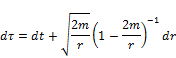Solving this for dt and substituting the result into Hilbert’s solution finally gives the Painlevé-Gullstrand ‘extension’,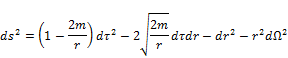(5)

This metric is not singular at r = 2m. It seems that it is singular only at r = 0. The cosmologists proclaim and celebrate removal of the singularity at the ‘Schwarzschild radius’ r = 2m, with a continuous descent to r = 0. Note however, that when r < 2m the sign of the coefficient of 2 still changes from + to −. The first two terms in Hilbert's solution change their signs from ± to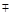respectively when r < 2m. Neither Schwarzschild’s solution nor Droste’s solution permits change of signs anywhere. The radius of the black hole event horizon is still, say the cosmologists, at their ‘Schwarzschild radius’ r = 2m, when the coefficient of 2 is zero. Their physical singularity, they say, is still at r = 0.

Applying the same method to Schwarzschild’s solution yields,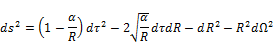(6)

This metric seems to be singular only at r = −α. It looks like the Painlevé-Gullstrand method has successfully driven Schwarzschild's solution down to r = −α. Applying the same method to Droste’s solution yields,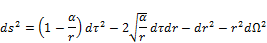(7)

This metric seems to be singular only at r = 0. It looks like the Painlevé-Gullstrand method has successfully driven Droste’s solution down to r = 0. Hilbert’s solution is nothing but Droste’s solution augmented with an apparent extension thereof and with α = 2m.

But appearances are often deceiving, and so it is here, as the next section reveals.

5. The Solution Ground-Form

Recall from Section 2 that Hilbert’s r can be replaced by any analytic function of r without violating spherical symmetry or Rμν = 0, yet not any analytic function of r is permissible. Every element of the necessary infinite equivalence class must satisfy the following conditions:

1.   it must be static

2.   it must be spherically symmetric

3.   it must satisfy Rμν = 0

4.   it must be asymptotically flat.

Condition (1) is actually redundant since conditions (2) and (3) ensure it, although it is not obvious. Nevertheless these are the conditions that Schwarzschild applied, following Einstein’s prescription. The infinite equivalence class that satisfies these conditions is [1-5],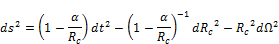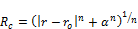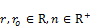(8)

Here the constants ro and n are entirely arbitrary. Setting ro = 0, n = 3, ror, produces Schwarzschild’s solution. Setting ro = α, n = 1, ror, produces Droste’s solution. Setting ro = 0, n = 1, ror, produces Brillouin’s solution . Setting ro = α/2, n = 1, ror, (and α = 2m) produces what cosmologists call the Harmonic radial coordinate (except that according to the cosmologists, 0 ≤ r, as is their want ). Hilbert’s solution is not an element of the infinite equivalence class. Since equations (8) generate an infinite equivalence class, if any one element of the class cannot be extended then none can be extended. Set ro = 0 and n = 2. This yields,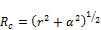(9)

This metric is singular only at r = 0. If this solution is extendible to produce a black hole, it requires that −α2r2. However, since r is a real number, r2 ≥ 0: the square of a real number can never have values less than zero, and so the theory of black holes violates the rules of pure mathematics . Hence (9) cannot be extended. Therefore none can be extended, on account of equivalence. The constant α appears in the expression for Rc in (8) owing to the radius Rp, which is given by [1,2],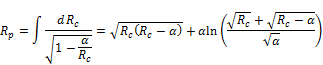Note that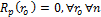.

Applying the Painlevé-Gullstrand method to the infinite equivalence class generator yields,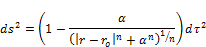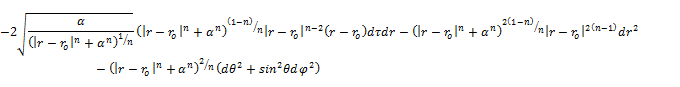(10)

or compactly as,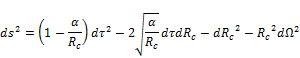(10b)

It is immediately clear that none can be extended to produce a black hole because the absolute value |r - ro|n can never have values less than zero, just as in the case of ro = 0, n = 2. Therefore, none of the elements of the infinite equivalence class can be extended, and so neither Schwarzschild’s solution nor Droste’s solution can be extended to produce a black hole. Yet it is by the alleged extension of Droste’s solution to Hilbert’s solution with α = 2m that the cosmologists first invented their black hole. In order to obtain Droste’s solution, set n = 1, ro = α, ro r in equations (10). The result is,(7b)

This is the same as expressions (7) except that α r. Metric (7b) is not ‘extended’ to Hilbert’s 0 ≤ r since the very choice of the arbitrary constants n and ro in expressions (10) preclude it. It is not necessary to always specify ror. For example, set n = 1, ro = α, rro. Then expressions (10) yield,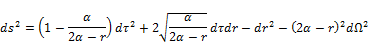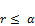(7c)

If expressions (7c) are mystically interpreted in the fashion of the cosmologists, then their black hole event horizon ‘Schwarzschild radius’ is at rs = α = 2Gm/c2 and their physical singularity is at r = 2α = 4Gm/c2. But rα by (7c). On the other hand, if it is assumed that r is the radius, in the fashion of the cosmologists, and therefore 0 ≤ r, then by the metric of (7c), their physical singularity is encountered at r = 2α = 4Gm/c2 before encountering their ‘event horizon’ at r = α = 2Gm/c2, nothing happens at r = 0, and r is never greater than 2α owing to the radial term. The foregoing amplifies the fact that r is not the radius, or even a distance, in any of the purported black hole solutions, contrary to the assertions of the cosmologists, and so their ‘Schwarzschild radius’ is not the radius of anything in Hilbert’s solution. Thus, the Painlevé-Gullstrand ‘coordinates’ do not produce a black hole. The Painlevé-Gullstrand extension is a delusion. Therefore the black hole is a fallacy.

6. The Irrelevance of Rμν = 0

Not only does the Painlevé-Gullstrand extension violate the rules of pure mathematics, Rμν = 0 and its solution have no physical counterpart, and so the black hole is again a fallacy. The reason for this is simple - material sources of a gravitational field cannot be both present and absent by means of the very same mathematical constraint in Einstein’s field equations. Consider Einstein’s field equations, with the so-called ‘cosmological constant’ λ included: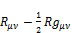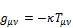(11)

Recall that according to Einstein his gravitational field is spacetime curvature induced by the presence of matter. Matter is the cause of Einstein’s gravitational field. Moreover, according to Einstein everything except his gravitational field is matter and the material sources of his gravitational field are described by his energy-momentum tensor Tμν.

Einstein and his followers assert that if λ = 0 and Tμν = 0, equations (11) reduce to,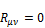(12)

These vacuum field equations, they claim, describe Einstein’s gravitational field outside a body such as a star. The cosmologists go further and profess that they also describe a black hole. Note that the gravitational field outside a body such as a star or black hole has a single causative material source: the mass of the star or black hole. Indeed, Einstein  asserted in relation to the solution to (12),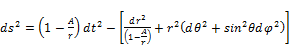(109a)M denotes the sun’s mass centrally symmetrically placed about the origin of co-ordinates; the solution (109a) is valid only outside this mass, where all the Tμν vanish.''

According to McMahon ,

"The vacuum field equations describe the metric structure of empty space surrounding a massive body. … r = 2m. This value is known as the Schwarzschild radius. In terms of the mass of the object that is the source of the gravitational field, it is given by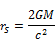Thus, although Tμν = 0, a material source is allegedly present. However, Einstein and his followers also assert that if λ ≠ 0 and Tμν = 0, equations (11) reduce to,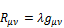(13)

The solution for this set of equations is de Sitter’s empty universe, which is empty because it contains no matter: Tμν = 0, is precisely why it contains no matter.

Ÿ "the de Sitter line element corresponds to a model which must strictly be taken as completely empty." Tolman 

Ÿ "This is not a model of relativistic cosmology because it is devoid of matter." d’Inverno 

Ÿ "the solution for an entirely empty world." Eddington 

Ÿ "there is no matter at all!" Weinberg 

Thus, by equations (12) and (13) Einstein and his followers assert that material sources are both present and absent by the very same mathematical constraint for material sources. This is impossible, because it is a contradiction . Rμν = 0 contains no material sources for the very same reason that Rμν = λgμν contains no material sources. Therefore the solution for Rμν = 0 contains no material sources, Rμν = 0 has no physical meaning, and so the black hole is fallacious.

Consequently, if Tμν = 0, not only are there no material sources present, and hence no gravitational field, there is no universe! This is amplified by writing equations (11) in the following equivalent forms and comparing,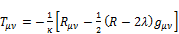(14)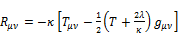(15)

Then according to equations (14), if Rμν = 0 then −κTμν = λgμν. The latter is a meaningless expression, unless both Tμν = 0 and λ = 0. According to equations (15), when Tμν = 0 then Rμν = λgμν. The latter leads to de Sitter’s empty universe, which bears no relation to reality, and so it too is a meaningless expression, unless Rμν = 0 and λ = 0. Thus, when either Tμν = 0 or Rμν = 0 then all other terms are also zero (they vanish identically), so that the identity 0 = 0 obtains . This means that if Tμν = 0 then not only is there no gravitational field, since there is no material source present to cause it, there is no universe.

If λ = 0 then equations (14) and (15) reduce to Einstein’s original form,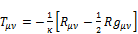(16)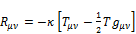(17)

According to equation (16) if Rμν = 0 then Tμν = 0, and by equation (17) if Tμν = 0 then Rμν = 0, the latter being physically meaningless. Thus, Rμν and Tμν must vanish identically to yield 0 = 0. If there is no material source to produce a gravitational field not only is there no gravitational field, there is no universe. In any event, the black hole is a fallacy.

7. Other Physical Impossibilities

According to the cosmologists their black hole has a finite mass and this mass is concentrated at a physical singularity at r = 0 in Hilbert’s solution, where volume is zero, density is infinite, and spacetime is infinitely curved. Gravity is not a force in General Relativity because it is spacetime curvature. Thus, the physical singularity of a black hole produces infinite gravity. However, no finite mass can have zero volume, have infinite density, or produce infinite gravity anywhere [2,20].

The cosmologists unwittingly adorn their black hole with the contradictory properties of having and not having an escape speed simultaneously at their ‘event horizon’. However, it is in fact impossible for anything to have and not have an escape speed simultaneously at the same place [2,21]. No cosmologist even understands the meaning of escape speed.

8. Isotropic Coordinates

A similar analysis of the solution for Rμν = 0 in the so-called ‘isotropic coordinates’ again proves the black hole is a fallacy [2,22]. The solution ground-form for the required infinite equivalence class in isotropic coordinates4 is,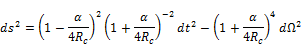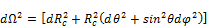wherein ro and n are arbitrary constants. There is no possibility for the fabled black hole.

The constant α appears in Rc due to the radius Rp, which is given by [2,22],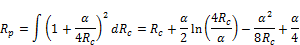Note that. Of course, the isotropic form of Schwarzschild spacetime is also physically meaningless anyhow.

9. Conclusions

The reasons why cosmologists have produced and perpetuated black holes are simple:-

a)  They incorrectly assume with Hilbert that r in Hilbert’s solution is the radius.

b)  Along with Hilbert, on account of (a), they erroneously think that 0 ≤ r in Hilbert’s metric, to produce thereby singularities at both r = 2m and at r = 0.

c)   Ignorant of the necessary infinite equivalence class they unwittingly use and abuse one specific element of the equivalence class to produce erroneous ‘extensions’ thereof.

d)  They insinuate the Newtonian expression for escape speed into Hilbert’s solution: an implicit 2-body relation into what is alleged to be a solution for a 1-body universe, in order to satisfy the false claim that Rμν = 0 describes Einstein’s gravitational field outside a body such as a star or black hole.

e)   Along with Einstein, they unwittingly invoke the very same mathematical constraint to have material sources both present in and absent from Einstein’s field equations.

f)   On account of the foregoing they unwittingly violate the rules of pure mathematics, invoke impossible physical properties, and reify mathematical entities.

g)   By wishful thinking and concomitant mass-media induced mass-hysteria, they garner the suspension of disbelief at large.

Just as people who believe in ghosts assign the action of ghosts to that which they do not understand, cosmologists assign the action of black holes to that which they do not understand. It is no wonder that these ‘peer reviewed’ cosmologists, some with a Nobel Prize, also assert that ‘near infinite’ is a scientific quantity [2,23], multiply infinity by 2 to make it double in size , and are now, with a large pot of money at their disposal, scouring the Cosmos for aliens . The proclivity of the Human Condition to readily embrace such irrational notions is well documented by anthropologists . The intellectual decrepitude of modern physics and astronomy is clear indication that they are diseased and dying sciences .

References

1. Crothers, S. J., On the General Solution to Einstein’s Vacuum Field and Its Implications for Relativistic Degeneracy, Progress in Physics, v.1, pp.68-73, 2005, http://www.ptep-nline.com/index _files/2005/PP-01-09.PDF.
2. Crothers, S. J., General Relativity: In Acknowledgement Of Professor Gerardus ‘t Hooft, Nobel Laureate, http://vixra.org/pdf/1409.0072v7.pdf.
3. Crothers, S. J., On the Generation of Equivalent ‘Black Hole’ Metrics: A Review, American Journal of Space Science, 2015, http://thescipub.com/abstract/10.3844/ofsp.9977, http://vixra.org/pdf/1507.0098v1.pdf.
4. Crothers, S. J., On the ‘Stupid’ paper by Fromholz, Poisson and Will, http://vixra.org/pdf/1310.0202.pdf.
5. Crothers, S. J., A Few Things You Need to Know to Tell if a Mathematical Physicist is Talking Nonsense: theBlack Hole - a Case Study, 29 July, 2015, http://vixra.org/pdf/1508.0007v1.pdf.
6. Schwarzschild, K., On the Gravitational Field of a Point Mass According to Einstein's Theory, Sitzungsber. Preuss. Akad. Wiss., Phys. Math. Kl: 189 (1916), http://arxiv.org/pdf/physics/9905030v1.
7. Droste, J., The field of a single centre in Einstein's theory of gravitation, and the motion of a particle in that field, Ned. Acad. Wet., S.A., v. 19, 197, 1917, http://www.dwc.knaw.nl/DL/publications/PU00012346.pdf.
8. Abrams, L. S., Black holes: the legacy of Hilbert's error, Can. J. Phys., v. 67, 919, 1989, http://arxiv.org/abs/gr-qc/0102055.
9. Dictionary of Geophysics, Astrophysics, and Astronomy, Matzner, R. A., Ed., CRC Press LLC, Boca Raton, LA, 2001, http://www.4shared.com/office/DYuEHhd3/dictionary _of _geophysics_astro.html.
10. Brillouin, M., The singular points of Einstein's Universe. Journ Phys. Radium, v. 23, 43, 1923, www.sjcrothers.plasmaresources.com/brillouin.pdf.
11. Fromholz, P., Poisson, E., Will, C., The Schwarzschild metric: It's the coordinates, stupid! 2 Aug. 2013, arXiv:1308.0394v1 [gr-qc].
12. Crothers, S. J., A Few Things You Need to Know to Tell if a Mathematical Physicist is Talking Nonsense: the Black Hole - a Case Study, 29 July, 2015, http://vixra.org/pdf/1508.0007v1.pdf.
13. Einstein, A., The Meaning of Relativity, expanded Princeton Science Library Edition, 2005.
14. McMahon, D., Relativity Demystified, A Self teaching Guide, McGraw-Hill, New York, 2006.
15. Tolman, R. C., Relativity Thermodynamics and Cosmology, Dover Publications Inc., New York, 1987.
16. d’Inverno, R., Introducing Einstein’s Relativity, Oxford University Press, 1992.
17. Eddington, A. S., The mathematical theory of relativity, Cambridge University Press, Cambridge, 2nd edition, 1960.
18. Weinberg, S., Gravitation and Cosmology: Principles and Applications of the General theory of Relativity, John Wiley & Sons, Inc., 1972.
19. Crothers, S. J., To Have and Not to Have - the Paradox of Black Hole Mass, 12 August, 2015, http://vixra.org/pdf/1508.0106v1.pdf.
20. Crothers, S. J., A Few Things You Need to Know to Tell if a Nobel Laureate is Talking Nonsense, 10 July 2015, http://vixra.org/pdf/1507.0067v2.pdf.
21. Crothers, S. J., Black Hole Escape Velocity - a Case Study in the Decay of Physics and Astronomy, http://vixra.org/pdf/1508.0066v1.pdf.
22. Crothers, S. J., On Isotropic Coordinates and Einstein’s Gravitational Field, Progress in Physics, v.3, pp.7-12, 2006, http://www.ptep-online.com/index_files/2006/PP-06-02.PDF.
23. Crothers, S. J., A Nobel Laureate Talking Nonsense: Brian Schmidt - a Case Study, 16 July, 2015, http://vixra.org/pdf/1507.0130v1.pdf.
25. Frazer, J. G., The Golden Bough, (A new abridgement), Oxford University Press, Oxford, 2009.
26. Robitaille, P.-M., Crothers, S. J., "The Theory of Heat Radiation" Revisited: A Commentary on the Validity of Kirchhoff’s Law of Thermal Emission and Max Planck’s Claim of Universality, Progress in Physics, v. 11, p.120-132, 2015, http://www.ptep-online.com/index_files/2015/PP-41-04.PDF.

Footnotes

 Here the speed of light is set to unity; c = 1.

 The speed of light is again unity; c = 1.

 Although the cosmologists give r many different and erroneous identities, they always treat it as the radius, as the ‘Schwarzschild radius’ alone attests; [2, 9].

 Once again, c = 1.

 Contents 1. 2. 3. 4. 5. 6. 7. 8. 9.
Article ToolsAbstractPDF(243K)